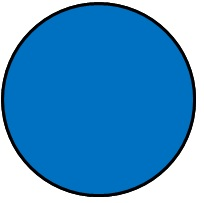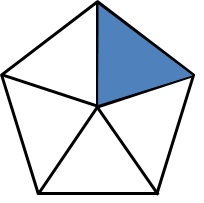Chapter 7 Class 7 Comparing Quantities
Concept wise

We often hear things like,

‘He topped the class with 95% marks’

‘The air around us has 21% of oxygen’

or       ‘This cloth is made up of 80% of cotton’

But what exactly do we mean by this?

Percentage is per-cent

where, cent  ⇒ century  = 100

Hence, percentage is per 100

So, percent (%) = 1/100

What does 50% mean?50% means 50 out of 100

i.e.

50% = 50 × 1/100

= 50/100

= 5/10

= 1/2

So, 50% means 1/2

#### What does 100% mean?100% means 100 out of 100

100% = 100 × 1/100

= 1

So, 100% means 1

#### What does 20% mean?20% means 20 out of 100

20% = 20 × 1/100

= 20/100

= 2/10

= 1/5

So, 20% means 1/5

We can also  represent percentage in decimals.

#### Represent 50% in decimal

50% = 50 × 1/100

= 5 × 1/10

= 5/10

= 0.5

So, 50% also means 0.5

Represent 100 % in decimal

100% = 100 × 1/100

= 1

So, 100% also means 1

Represent 20 % in decimal

20% = 20 × 1/100

= 2 × 1/10

= 2/10

= 0.2

So, 20% also means 0.2

Learn in your speed, with individual attention - Teachoo Maths 1-on-1 Class